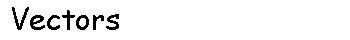3. Addition and subtraction of vectors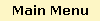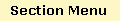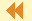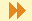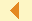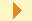GlossaryExamplesEngineering ExamplesQuiz### The triangle rule for addition

Page 3 of 7

Another way to define addition of two vectors is by a head-to-tail construction that creates two sides of a triangle. The third side of the triangle determines the sum of the two vectors, as shown below.

Place the tail of the vector v at the head of the vector u. That is, u =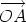and v =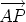.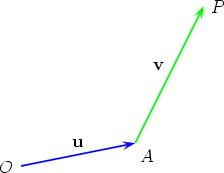Now construct the vector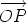to complete the third side of the triangle OAP.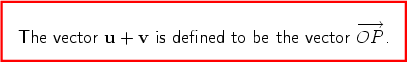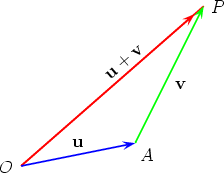This method is equivalent to the parallelogram law of addition, as can be easily seen by drawing a copy of v tail-to-tail with u, to obtain the same parallelogram as before.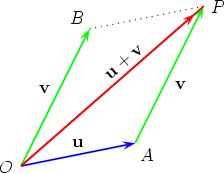Using position vector notation, the triangle rule of addition is written as follows: for any three points X, Y , Z,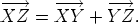Both the triangle and the parallelogram rules of addition are procedures that are independent of the order of the vectors; that is, using either rule, it is always true that u + v = v + u for all vectors u and v. This is known as the commutative law of addition. There are other rules like this one, and they are discussed in the component Vector Algebra.

Feedback